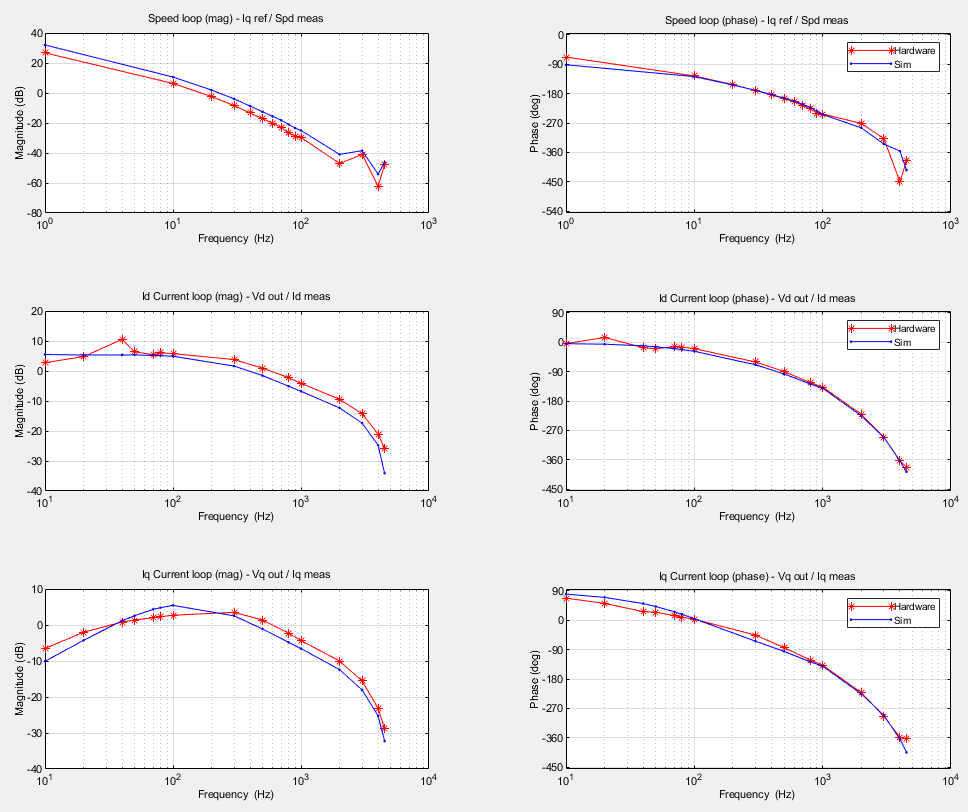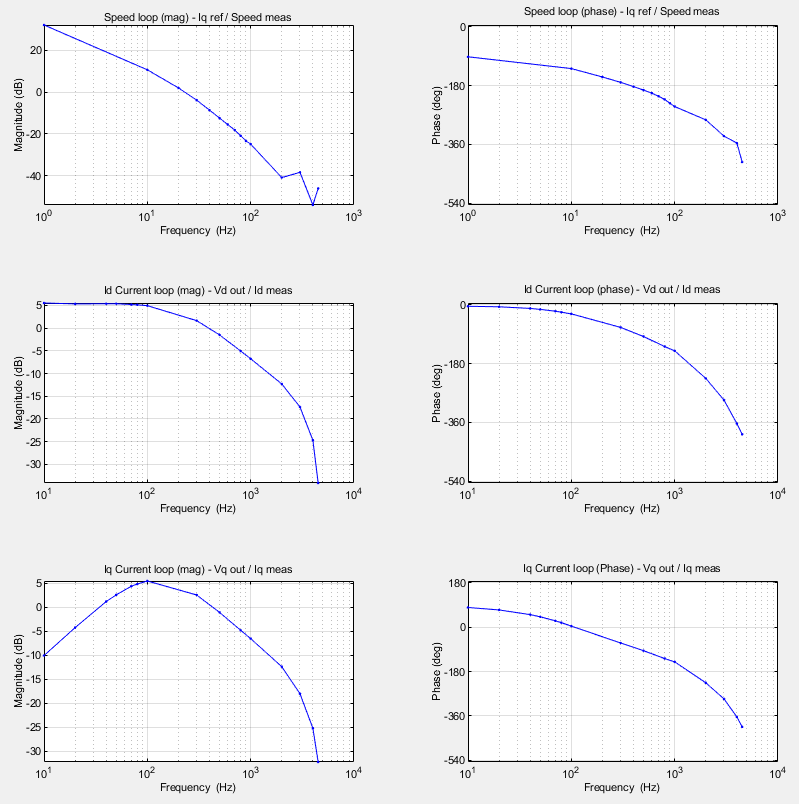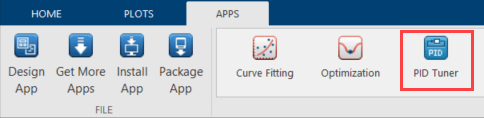# Frequency Response Estimation of PMSM Using Field-Oriented Control

This example performs frequency response estimation (FRE) of a plant model running a three-phase permanent magnet synchronous motor (PMSM). When you either simulate or run the model on the target hardware, it runs tests to estimate the frequency response as seen by each PI controller (also known as raw FRE data) and plots the FRE data to provide a graphical representation of the plant model dynamics.

When the motor runs in a steady state, the online Frequency Response Estimator block that is connected to each PI control loop (Id current, Iq current, and speed) sequentially perturbs the PI controller output and estimates the frequency response of the plant model as seen by each PI controller. You can use the frequency response of the plant to estimate the PI controller gains.The model uses the field-oriented control (FOC) technique to control the PMSM. The FOC algorithm requires rotor position feedback, which is obtained by a quadrature encoder sensor. For details about FOC, see Field-Oriented Control (FOC).

### Models

The example includes the model (target model) mcb_pmsm_freq_est_f28379d.

You can use this model for both simulation and code generation. You can also use the open_system command to open the model.

```open_system('mcb_pmsm_freq_est_f28379d.slx'); ```For details regarding the supported hardware configuration, see the Required Hardware topic in the Generate Code and Deploy Model to Target Hardware section.

### Required MathWorks® Products

To simulate model:

• Motor Control Blockset™

To generate code and deploy model:

1. Motor Control Blockset™

2. Embedded Coder®

3. Embedded Coder® Support Package for Texas Instruments™ C2000™ Processors

### Prerequisites

1. Obtain the motor parameters. We provide default motor parameters with the Simulink® model that you can replace with the values from either the motor datasheet or other sources.

However, if you have the motor control hardware, you can estimate the parameters for the motor that you want to use, by using the Motor Control Blockset parameter estimation tool. For instructions, see Estimate Motor Parameters Using Motor Control Blockset Parameter Estimation Tool.

The parameter estimation tool updates the `motorParam` variable (in the MATLAB® workspace) with the estimated motor parameters.

2. If you obtain the motor parameters from the datasheet or other sources, update the motor parameters and inverter parameters in the model initialization script associated with the Simulink® models. For instructions, see Estimate Control Gains from Motor Parameters.

If you use the parameter estimation tool, you can update the inverter parameters, but do not update the motor parameters in the model initialization script. The script automatically extracts motor parameters from the updated `motorParam` workspace variable.

### Simulate Model

This example supports simulation. Follow these steps to simulate the model.

1. Open the target model included with this example.

2. Click Run on the Simulation tab to simulate the model.

3. Click Data Inspector on the Simulation tab to view and analyze the simulation results.

4. On the target model, click the Plot freq response hyperlink to plot the frequency response data of the plant model (`sys_sim_id`, `sys_sim_iq`, and `sys_sim_spd` variables in the workspace) that the speed control loop and the current control loops measure.

### Generate Code and Deploy Model to Target Hardware

This section instructs you to generate code, run the FOC algorithm on the target hardware, start frequency response estimation, and plot the FRE data.

This example uses a host and a target model. The host model is a user interface to the controller hardware board. You can run the host model on the host computer. The prerequisite to use the host model is to deploy the target model to the controller hardware board. The host model uses serial communication to command the target Simulink® model and run the motor in a closed-loop control.

Required Hardware

This example supports this hardware configuration. You can also use the target model name to open the model from the MATLAB® command prompt.

NOTE: When using the BOOSTXL-3PHGANINV inverter, ensure that proper insulation is available between bottom layer of BOOSTXL-3PHGANINV and the LAUNCHXL board.

For connections related to the preceding hardware configurations, see LAUNCHXL-F28069M and LAUNCHXL-F28379D Configurations.

Generate Code and Run Model on Target Hardware

1. Simulate the target model and observe the simulation results.

2. Complete the hardware connections.

3. The model automatically computes the ADC (or current) offset values. To disable this functionality (enabled by default), update the value `0` to the variable `inverter.ADCOffsetCalibEnable` in the model initialization script.

Alternatively, you can compute the ADC offset values and update it manually in the model initialization scripts. For instructions, see Run 3-Phase AC Motors in Open-Loop Control and Calibrate ADC Offset.

4. Compute the quadrature encoder index offset value and update it in the `pmsm.PositionOffset` variable available in the model initialization script associated with the target model. For instructions, see Quadrature Encoder Offset Calibration for PMSM Motor.

5. Open the target model for the hardware configuration that you want to use. If you want to change the default hardware configuration settings for the model, see Model Configuration Parameters.

6. Load a sample program to CPU2 of LAUNCHXL-F28379D, for example, program that operates the CPU2 blue LED by using GPIO31 (`c28379D_cpu2_blink.slx`), to ensure that CPU2 is not mistakenly configured to use the board peripherals intended for CPU1.

7. Click Build, Deploy & Start on the Hardware tab to deploy the target model to the hardware.

8. Click the host model hyperlink in the target model to open the associated host model. You can also use the open_system command to open the host model.

```open_system('mcb_pmsm_freq_host_f28379d.slx'); ```For details about the serial communication between the host and target models, see Host-Target Communication.

9. In the Host Serial Setup block mask of the host model, select a Port name.

10. Change the position of the Start / Stop Motor switch to On, to start running the motor.

11. Update the Reference Speed value in the host model.

12. Select the debug signal that you want to monitor in the Debug signals section of the host model. Observe these signals in the SelectedSignals time scope.

13. Click the FRE Trigger button to start the FRE process on the target hardware.

14. Select Position & Raw FRE data in the Debug signals section of the host model to start receiving the raw FRE data from the target hardware. The FRE Status LED turns amber to indicate that the host model is receiving raw FRE data from the target hardware.

NOTE: The LED shows the correct status only when you select Position & Raw FRE data in the Debug signals section. Otherwise, the LED remains grey.

15. Check the status of the FRE Status LED on the host model. The LED turns green after the host model receives all the raw FRE data from the target hardware.

16. Click the FRE Plot button to plot the raw FRE data received from the target hardware.

17. On the host model, click Stop on the Simulation tab to stop the simulation.

18. Click the compare hyperlink in the host model to plot the raw FRE data generated during simulation and hardware run together and compare them.

For an accurate comparison, we recommend that you use the same reference speed during simulation and when running the example on the target hardware.NOTE:

• To stop the FRE process any time, click the FRE Abort button.

• To stop the motor immediately, turn the Start / Stop Motor switch Off.

### Configure Frequency Response Estimator Block

Configure these parameters in the Frequency Response Estimator block (from Simulink Control Design™ toolbox) mask:

• Sample time (Ts) - Enter a block sample time that is identical to that of the PI controller.

• Frequencies - Enter an array of frequencies at which the block perturbs the PI controller output to estimate the frequency response of the plant. This field uses the (single data type) workspace variable `fre.i_freq` to store the array of perturbation signal frequencies.

NOTE: By default, the model uses an array size of fifteen. However, you can configure the array size.

The start/stop signal value of `1` that started the FRE experiment should change to `0` only after the perturbations and tests for all the frequencies are complete and the FRE experiment ends.

• Amplitudes - Enter the amplitude of the perturbation signals that the block applies to the PI controller output to estimate the frequency response of the plant. This field uses the (single data type) workspace variable `fre.i_amp` to store the common amplitude value of the perturbation signals.

A high amplitude produces disturbances when the motor runs. An amplitude that is too low results in an inaccurate FRE.

For more details about the Frequency Response Estimator block, see Frequency Response Estimator (Simulink Control Design).

### Frequency Response Estimator Block Output

The Frequency Response Estimator block (connected to each PI controller) performs an FRE experiment by perturbing the PI controller output using the sequence of frequencies stored in `fre.i_freq`.

For each perturbation signal (represented by a frequency) the block estimates the plant frequency response in the form of a complex number. Therefore, block uses the array of frequencies to generate an array of complex numbers (raw FRE data).

The generated sequence of complex numbers contains the information related to gain and phase delay.

### Controlling FRE Experiments

The State Machine Control subsystem algorithm enables the three Frequency Response Estimator blocks one at a time (and therefore, runs the three FRE experiments) in this order by using the start/stop input port of the Frequency Response Estimator block:

1. FRE block connected to Id control loop

2. FRE block connected to Iq control loop

3. FRE block connected to speed control loop

The state machine control ensures that the time interval between the start and stop signals is greater than or equal to the FRE experiment length (as displayed by the Frequency Response Estimator block dialog). Therefore, if you change the perturbation signal frequencies, ensure that the state machine control sends the stop signal only after the FRE experiment ends.

For more details about the Frequency Response Estimator block, see Frequency Response Estimator (Simulink Control Design).

### Plot Frequency Response After Simulation

After the simulation ends, the target model stores the frequency response (or the raw FRE data) in these workspace variables:

• `out.Idfreqdata`- raw FRE data for the Id current PI controller.

• `out.Iqfreqdata` - raw FRE data for the Iq current PI controller.

• `out.Spdfreqdata` - raw FRE data for the speed PI controller.

When you click the Plot freq response hyperlink on the target model, it plots the frequency response for the three PI controllers.The target model uses these commands to plot the frequency responses as seen by the three PI controllers:

Frequency response of Id current PI controller:

`sys_sim_id = frd(out.Idfreqdata,fre.i_freq*2*pi);`

`bode(sys_sim_id);`

Frequency response of Iq current PI controller:

`sys_sim_iq = frd(out.Iqfreqdata,fre.i_freq*2*pi);`

`bode(sys_sim_iq);`

Frequency response of speed PI controller:

`sys_sim_spd = frd(out.Spdfreqdata,fre.spd_freq*2*pi);`

`bodeplot(sys_sim_spd);`

• `mcb_pmsm_freq_est_plot.m`

• `mcb_pmsm_freq_host_est_plot.m`

### Send Raw FRE Data to Host Model

When running the target model on the hardware, it transfers the raw FRE data continuously to the host model.

The target model splits the entire sequence of the complex numbers (or raw FRE data) from each FRE block into real and imaginary arrays and adds headers to separate them. It uses this format to send the raw FRE data from each FRE block to the host model by using serial communication.### Plot Frequency Response When Using Target Hardware

After receiving the message from the target hardware, the host model decrypts the message and stores the array of complex numbers (raw FRE data) in these workspace variables:

• `IdfreqData` - raw FRE data for the Id current PI controller.

• `IqfreData` - raw FRE data for the Iq current PI controller.

• `SpdfreqData` - raw FRE data for the speed PI controller.

When you click the FRE Plot button, the host model plots the frequency response for the three PI controllers.The host model uses these commands to plot the frequency responses observed for the three PI controllers:

Frequency response of Id current PI controller:

`sys_hw_id=frd(IdFreqData.signals.values,fre.i_freq*2*pi);`

`bode(sys_hw_id);`

Frequency response of Iq current PI controller:

`sys_hw_iq=frd(IqFreqData.signals.values,fre.i_freq*2*pi);`

`bode(sys_hw_iq);`

Frequency response of speed PI controller:

`sys_hw_spd=frd(SpdFreqData.signals.values,fre.spd_freq*2*pi);`

`bode(sys_hw_spd);`

• `mcb_pmsm_freq_est_plot.m`

• `mcb_pmsm_freq_host_est_plot.m`

### Tuning PI Controller Gains

These steps describe how to tune and determine the gains for the Id current, Iq current, and speed PI controllers:

1. Navigate to Simulink tool strip > Apps and open the PID Tuner app.2. In the PID Tuner tab, select PI for Type, Parallel for Form, and Frequency for Domain.3. In the PID Tuner tab, select Plant > Import.4. In the Import Linear System window, select sys_sim_id and click Import to import the FRE data for the Id PI controller.5. Select Add Plot > Bode > Open-loop to open the open-loop bode plot for the Id PI controller.6. Use the Tuning Tools section in the PID Tuner tab to tune the bandwidth and phase margin and observe the results in the open-loop and plant bode plots.7. After completing tuning click Show Parameters to display the tuned controller parameters Kp and Ki for the Id current PI controller.8. Repeat steps 3 to 7 by selecting sys_sim_iq in the Import Linear System window to obtain the tuned parameters Kp and Ki for the Iq PI controller.

9. Update the Kp and Ki gain values for both Id and Iq current PI controllers in the initialization script of the example model `mcb_pmsm_freq_est_f28379d.slx`. For instructions, see Estimate Control Gains from Motor Parameters.

10. Perform the frequency response estimation again using the updated PI controller gains by either simulating the example or running it on the target hardware.

11. Perform steps 3 to 7 by selecting sys_sim_spd in the Import Linear System window to obtain the tuned parameters Kp and Ki for the speed PI controller.

### Other Things to Try

You can try estimating the transfer functions and state-space models from the FRE data by using these functions from the System Identification Toolbox™:

• ssest

• tfest

Get ebook